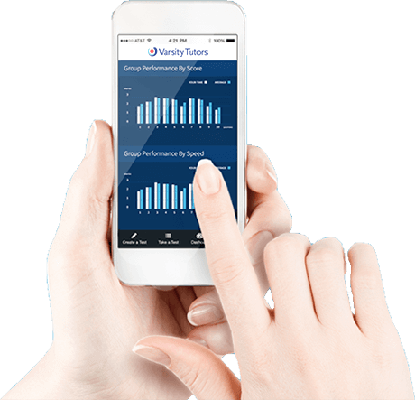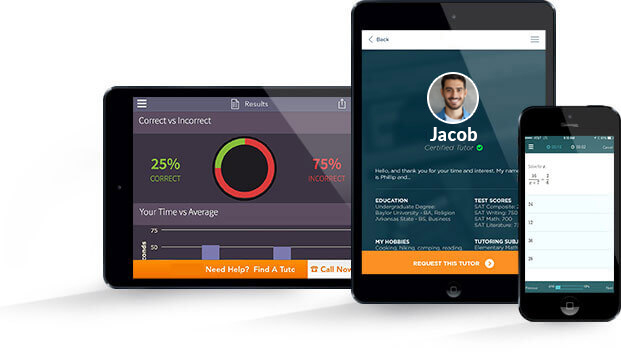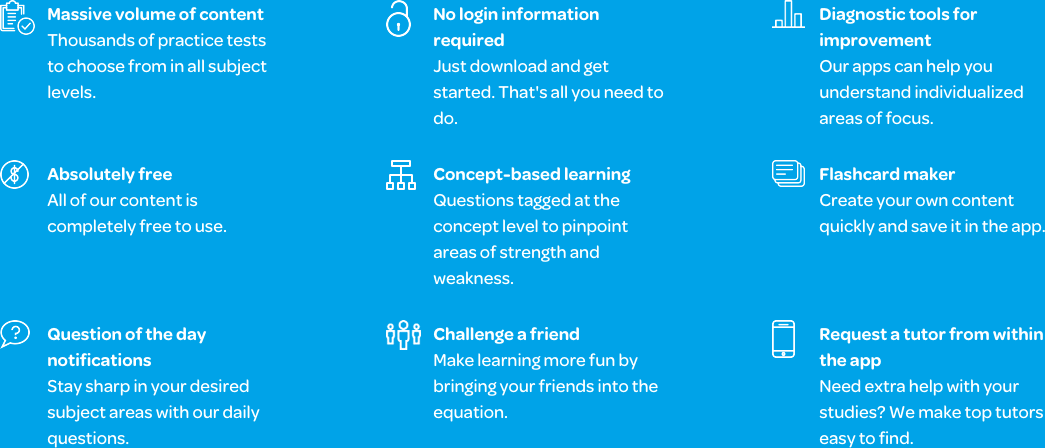# Varsity Tutors College Algebra mobile app### The Varsity Tutors College Algebra Mobile App

College Algebra can be a difficult subject. It requires you to be able to think abstractly as you solve linear and quadratic equations, polynomials, and logarithmic functions. It is also an important subject that many students are required to complete for their degree programs. If you are someone who is struggling with College Algebra, or you simply want to better understand the course, Varsity Tutors’ Learning Tools free College Algebra app can provide you the help you need to supplement your learning. It doesn’t matter if you are using an iPad, iPhone, or an Android device, you are a mere click away from getting the help you need to succeed.

When you study with the College Algebra mobile app, you will be able to supplement your learning in a manner that is convenient for you. You can select from a wide range of topics relating to College Algebra and are able to choose areas that best suit your needs. By doing this, you are working to prepare yourself for any upcoming tests while studying efficiently. Whether you need help covering exponential functions, working with graphs, or solving various equations, the College Algebra mobile app is the perfect program for you.

When you access the program, you will be able to choose from several different functions. The Question of the Day, for example, is an excellent way to help you as you review College Algebra daily. Here, you will be given a different question every day that you are expected to answer. This is a great way to give you the introduction you need to start thinking like a mathematician. It is also good for people who want to spend a short amount of time studying each day at their convenience.

Using the mobile app also gives you access to flashcards, which cover different topics related to College Algebra. When you study with the flashcards, you are able to work through a variety of specific areas of College Algebra, which is ideal if you want to focus on a particular area, or even create your own deck of cards to study with. In addition, you can also use the Learn by Concept feature and benefit from an in-depth course curriculum that allows you to study all of the subjects covered in a College Algebra course.

After you feel as if you’ve reviewed sufficiently, you will be able to take various practice tests. The mobile app offers short, subject-based practice tests that evaluate your knowledge on specific parts, as well as the Full-Length Practice Tests. These tests are much longer and cover everything taught in the course, and they are an ideal way to help you prepare for an upcoming test. At the end of each test you can see your results, read detailed explanations of how each correct answer was reached, or compare your score against other test taker’s scores.

No matter what your goals are, the College Algebra mobile app is an excellent way to help you get the assistance you need to succeed. The program is free and is available on iTunes or the Google Play Store. By spending a little bit of time each day studying with the program, you are working to get the assistance you need to help you pass your College Algebra courses.The first semester of college is often equal parts excitement and terror. Students are expected to navigate a new social and academic environment, choose a major which will help determine their life path, and decide the order of classes to take to achieve their goals. Depending on your major, College Algebra may be a course available for a math credit, or it may be a prerequisite for the courses on your degree plan. Either way, a comprehensive supplemental tool like Varsity Tutors’ College Algebra app for your iPhone, iPad, or Android device may be just the thing you need to put yourself in position to succeed.

The names given to college algebra classes can be so confusing. It is important to note there is a significant difference between College Algebra and Algebra for College Students. College Algebra immediately follows Algebra 2 and is to prepare students for Calculus. Typically, the first semester will include advanced algebraic concepts, and the second semester will include trigonometry concepts. Even more confusing for many students, the concepts covered in various algebra classes appear to be the same if one is looking at the Table of Contents for the book or the course syllabus. However, the depths of coverage increases exponentially along with the level. The concepts covered in College Algebra include a review of algebraic concepts covered in previous courses, exponential and logarithmic functions, graphs, polynomial functions, solving equations and inequalities, and systems of equations

There is not a single standardized exam for College Algebra. While testing procedures will vary by university, most courses have a cumulative midterm and final exam based on class lectures and information from the textbook. Typically, the course syllabus will detail how much of the exam will be pulled from the topics covered throughout the course. When you download the Varsity Tutors College Algebra app from iTunes or the Google Play Store, you can take free practice tests that will give you a chance to see if you are ready for test day.

College Algebra is designed to take your mastery of algebra to a point where you will be prepared to begin calculus. In order to ensure students are fully prepared for more advanced concepts, it is often necessary to review fundamental knowledge. Some of the topics that may be reviewed in this class include complex numbers, factoring polynomials, integer exponents, polynomials, radicals, rational exponents, rational expressions, and real exponents.

Exponential and logarithmic functions will be explored in greater depth in this class. They are both important components in theoretical and practical application. An exponential function is a function where the independent variable is an exponent. Conversely, logarithmic functions represent the inverse of exponential functions. You will learn to identify and solve exponential and logarithmic functions.

You will be working extensively with a graphing calculator in College Algebra. However, to do so effectively, it is necessary to understand the underlying mathematical principles and necessary equations. Graphs are significant in understanding and interpreting points and lines with algebraic equations. Equations can be used to find ellipses, hyperbolas, lines, circles, piecewise functions, miscellaneous functions, parabolas, rational functions, symmetry, and transformations on a graph.

The word polynomial comes from the word parts “poly-“ and “-nomial,” which mean “many” and “terms,” respectively. A polynomial can have constants, variables, and exponents. They can be combined using subtraction, addition, multiplication, and division, but they can never be divided by a variable. A polynomial function is a function such as a cubic, quadratic, or a quartic that involves only non-negative integer powers of x. Students will be engaged in dividing polynomials, finding zeros of a polynomial, graphing polynomials, and finding the roots of a polynomial. Sound difficult? You can review this and other topics comprehensively with Varsity Tutors’ free College Algebra app for iOS and Android devices to stay on top of your studies.

Equations and inequalities are broken down into systems of each. Systems of equations include solution sets designed to factor an equation, find a solution set, and find a solution for a system of equations. Linear, rational, and variable equations illustrate how to determine when there is no solution for an equation and how to find the solution to a rational equation. Quadratic equations cover how to factor and find the solution for quadratic equations. Systems of inequalities show how to find the solution to an inequality with addition, division, multiplication, or subtraction.

The concepts of functions and lines are broken down into algebraic functions, equations of lines, graphing, and sequences. Algebraic functions include finding a direct variation, inverse variation, the domain of a function, and how to use the quadratic function. Equations of lines include the midpoint formula, the equation and slope of parallel lines, the equation and slope of perpendicular lines, points and distance formula, and how to find the slope and equation of a line. Graphing techniques include how to graph a specific function, line, point, quadratic, two-step inequality, absolute value function, an exponential function, and ordered pair. Sequences include how to find consecutive integers, finding the answer to arithmetic sequences, finding the common differences in sequences, and finding the next and nth term in an arithmetic sequence.

A linear equation is an equation between two variables that can be plotted in a straight line on a graph. While working with these types of equations, you will learn to convert measurements, solve absolute value equations, solve one and two-step equations, learn to write expressions and equations, and work with percents and proportions in equations. A linear inequality contains one of the symbols of inequality and involves a linear function. While working with these types of equations, you will learn the absolute value of inequalities, how to graph inequalities, and how to write out inequalities. Systems of equations are when two or more equations are working together. Throughout the course of your College Algebra class, you will work with augmented matrices, linear systems with two and three variables, and nonlinear systems.

At the end of this course, you will have a broader understanding of the complexities of algebra and a firm foundation from which to begin studying calculus. Calculus concepts are unlikely to be introduced in this course. Instead, algebraic concepts that may not have been fully explored in previous courses will be covered in greater depth. For some students, this may represent the end of their required math coursework, while for others it will be a stepping stone to more advanced math and science concepts. Regardless, the work is both challenging and rewarding, and will help you better understand algebraic concepts as they relate to your specific field of study. To take steps to ensure you better understand the concepts of the course, download the free Varsity Tutors College Algebra app today from iTunes or the Google Play Store.